# Massive Pulley and Slope

Jazz

## Homework Statement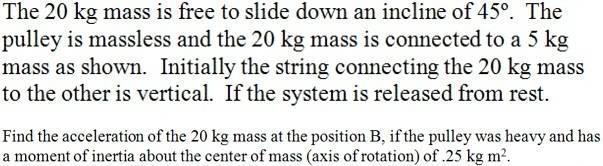NOTE: This is a problem I found and the question is actually the part B of it. That of ''massless pulley'' I suppose was dealing with the part A (which I don't know what question was), so please don't consider that (:.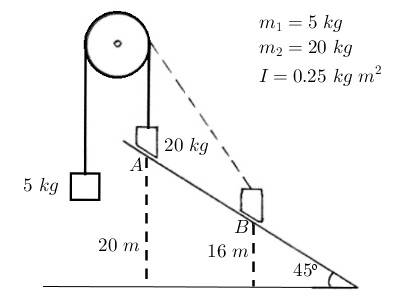## Homework Equations

##F_{net} = ma##
##\tau = I\alpha##

## The Attempt at a Solution

I followed this reasoning:

I started focusing on the pulley.

- The force acting on it are the tensions due to ##m_1## and that due to ##m_2##, so ##F_{net} = T_2 - T_1##.

- Since the pulley is massive, there is a torque there, so ##net\ \tau = I\alpha##. This torque is caused by the forces of tension acting on the pulley, then: ##T_2r - T_1r = I\alpha##.

- In this case ##T_1## is the pulling force exerted on ##m_1## to accelerate it upward: ##m_1g + m_1a##.
- ##T_2## is the pulling force exerted on ##m_2## to prevent it from accelerating at ##g##: ##m_2g - m_2a##.

- Replace this into the equation of torque:

##(m_2g - m_2a)r - (m_1g + m_1a)r = I\alpha##

- We know that ##\alpha = \frac{a}{r}##, so:

##(m_2g - m_2a)r - (m_1g + m_1a)r = I\frac{a}{r}##

And here I'm stuck. I have two unknowns: ##a## and ##r##. I suppose the two heights and the angle are given to be used to solve the problem but I don't know how.

The other thing I've been thinking is that, at ##B##, the vertical component of ##F_{net}## has two components: one perpendicular to the slope and the other parallel to it, but I don't know how to figure out the angle that the string makes with the ground in order to find the vertical component of ##F_{net}## and on the other hand, if I'm able to find it, the torque wouldn't be taken into account I guess.

Last edited:

Homework Helper
Gold Member
- ##T_2## is the pulling force exerted on ##m_2## to prevent it from accelerating at ##g##: ##m_2g - m_2a##.
How do you get that for T2? m1 and m2 do not have the same acceleration, m2's acceleration is not in the vertical direction, and you've omitted the normal force from the slope.

Jazz
How do you get that for T2? m1 and m2 do not have the same acceleration, m2's acceleration is not in the vertical direction, and you've omitted the normal force from the slope.

Here is my diagram: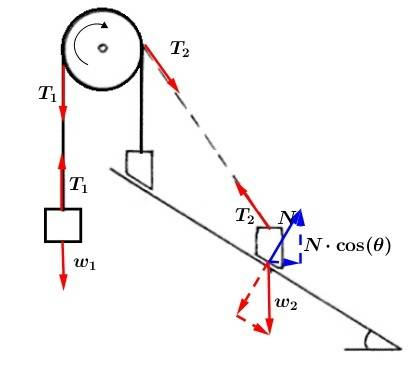True. From the fact that the pulley needs a net torque for it to rotate and hence that both tensions must not be in balance, the acceleration is not the same.

Well, I must start again. This is what I think:

- Forces on ##m_1##:

##F_1 = T_1 - w_1##

- Forces on ##m_2##:

Here I'm a little confused. It seems that I need to figure out the forces along the axes in order to find ##F_2##:

##F_{2(y)} = w_2 - (N\cdot \cos(\theta) + T_2\cdot \cos(\beta))##

##F_{2(x)} = N\cdot \sin(\theta) - T_2\cdot \sin(\beta)##

But I got that angle ##\beta## there |:

- Forces on the pulley:

##T_2r - T_1r = I\alpha##

I think there will be a ##F_{net}## on ##m_2## making it accelerate at ##\frac{F_{net}\cdot \sin(\theta)}{m_2}##, but I still don't know what I'm missing.

Last edited:
Homework Helper
Gold Member
Seems to me there's not enough information. There's nothing that fixes the height of the pulley above the ramp. You can easily work out the answer for two extreme case (1. rope parallel to ramp; 2. with the pulley at great height, the rope effectively vertical at all times) and see that the results are different.

Jazz
I would like to try this case: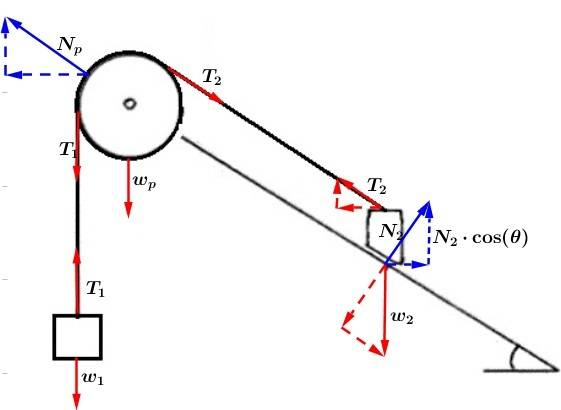In my new diagram the forces are:

- On ##m_1##:

##F_1 = T_1 - w_1##

- On ##m_2##:

##F_{2(y)} = w_1 - (N\cdot \cos(\theta) + T_2\cdot \sin(\theta))##

##F_{2(x)} = N_2\cdot \sin(\theta) - T_2\cdot \cos(\theta)##

- On the pulley:

##I\alpha = (T_2-T_1)r\hspace{35pt}(1)##

Trying to find the tensions:

##T_1 = w_1 + F_1##

##T_2 = w_2\cdot \sin(\theta) - T_1##

##T_2 = w_2\cdot \sin(\theta) - (w_1 + F_1)##

Replace into ##(1)##:

##I\alpha = (w_2\cdot \sin(\theta) - (w_1 + F_1)-(w_1 + F_1))r##

##I\frac{a}{r} = (w_2\cdot \sin(\theta) - 2(w_1 + F_1))r##

I have another ##a## within ##F_1##, but I still have that ##r## there that don't know how to get rid of.

Homework Helper
Gold Member
You need to bring the accelerations of the masses into it to get rid of F1, F2. The relationship between the angular and linear accelerations will get rid of the r.
You have a couple of typos above. Your first m2 equation references w1. Your last equation has an r on the right which should not be there.

Satvik Pandey
I would like to try this case:In my new diagram the forces are:

- On ##m_1##:

##F_1 = T_1 - w_1##

- On ##m_2##:

##F_{2(y)} = w_1 - (N\cdot \cos(\theta) + T_2\cdot \sin(\theta))##...........................(1)

##F_{2(x)} = N_2\cdot \sin(\theta) - T_2\cdot \cos(\theta)##......................................(2)

Hi, Jazz. I would like modify your eq(1) and eq(2). I will write ##F_{2(y)}## as ##m_{2}a_{2y}## and ##F_{2(x)}## as ##m_{2}a_{2x}##.

So ##m_{2}a_{2y}= w_1 - (N\cdot \cos(\theta) + T_2\cdot \sin(\theta))##

and ##m_{2}a_{2x}=N_2\cdot \sin(\theta) - T_2\cdot \cos(\theta)##.
I did it just to include the expression of accelerations.

Now we have to relation between ##a_{1}, ##a_{2y}## and ##a_{2x}## and ##\alpha##.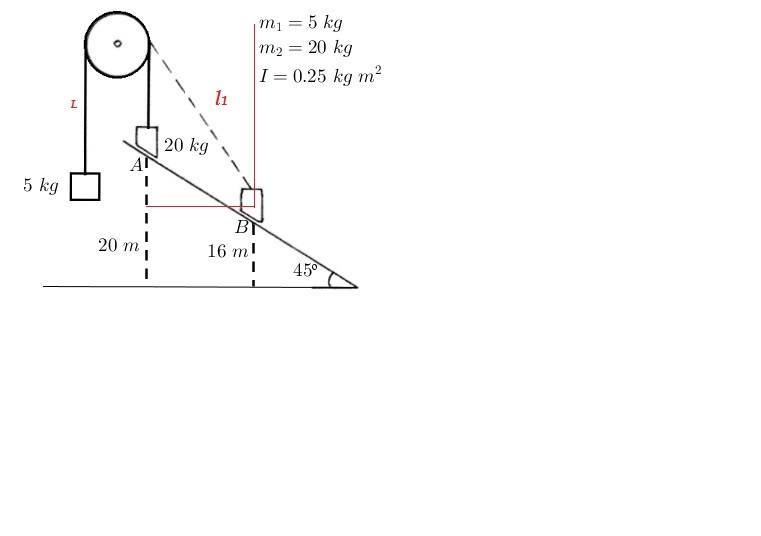Let ##L## be the distance between pulley and ##m_{1}## when the ##m_{2}## is at B. Let ##l_{1}## be the distance between ##m_{2}## and pulley. Now, can you find the horizontal and vertical displacement of ##m_{2}## from initial position in terms of ##l_{1}##? Differentiate the expressions twice wrt time.

Now in this type pf problem we assume that length of the string is constant. Let ##L_{0}## be the total length of the string.
So ##l_{1}+L=L_{0}##. What will you get if you differentiate this equation twice wrt time?

Use these two equation and find relation between ##a_{1}##, ##a_{2y}## and ##a_{2x}##.

As no information is given so I assume that the strings do not slip on the pulley. So from this assumption can you find relation between ##\alpha## and ##a_{1}##.